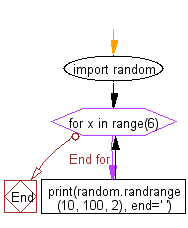﻿ Python Math: Generate random even integers in a specific numerical range - w3resource

# Python Math: Generate random even integers in a specific numerical range

## Python Math: Exercise-50 with Solution

Write a Python program to generate random even integers in a specific numerical range.

Sample Solution:

Python Code:

``````import random

for x in range(6):
print(random.randrange(10, 100, 2), end=' ')
```
```

Sample Output:

```74 54 12 58 18 82
```

Pictorial Presentation:Flowchart:Python Code Editor:

Have another way to solve this solution? Contribute your code (and comments) through Disqus.

What is the difficulty level of this exercise?

Test your Programming skills with w3resource's quiz.

﻿